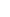34.1. LIGHT INTENSITY, DISTANCE AND (C+V) (C-V) MATHEMATICSIt is a well-known topic that light intensity changes depending on distance. As you move a circle from the light source, amount of light that passes through it in a unit of time decreases. The figure below shows this.The general rule is as follows: Intensity of a light that a point source emits around it decreases inversely proportional to the square of the distance. We can think that in practice when we double the distance, the light intensity will decrease fourfold. There is the following equation in line with this rule.Since Light Intensity is a result primarily based on the number of photons that make up the light, we can write the equation above by presenting the real reason as below:However, both equations above are valid for targets that are not in motion relative to light source. When there is movement, (c+v) (c-v) mathematics steps in the situation.We can see how (c+v) (c-v) mathematics interferes in the figure below. Think of an object that stands still at d0 distance to the light source. The fact that the object is drawn as a round shape is not important. Assume that n of the photons that are emitted at a time such as t0 choose this object as their arrival targets. As a result, n number of photons cover d0 distance in tΔ =d0/c time and reach their target objects. Now, lets think of the situation the object moves away. When the object is at d0 distance, n number of photons again choose the object as their arrival target and set out towards it. However, this time, the speed of the photons is (c+v) and these photons will arrive at the object at d1 distance instead of d0 distance and the number of photons will not change. In case the object comes to the light source, photons will reach the target, which travels at (c-v) speed, at d2 distance and the number of photons will, again, not change.The fact that the number of photons does not change doesnt mean that the object will get the same light intensity in all these cases because energies of the photons will change although their numbers stay the same.  The change in the energy will be directly proportional to wavelength change.  Therefore, we can define the equation: EX . λX = E0 . λ0. Then, an object that receives E0 amount of energy will receive E2= E0.c/(c+v) energy if it is in motion and if it is moving away, and it will receive E1= E0.c/(c-v) if it is approaching. In the figure, pay attention to the fact that d1 and d2 distances are determined by tΔ time. The number of photons not changing is directly related to tΔ time. The number of photons will change when the tΔ time is exceeded.